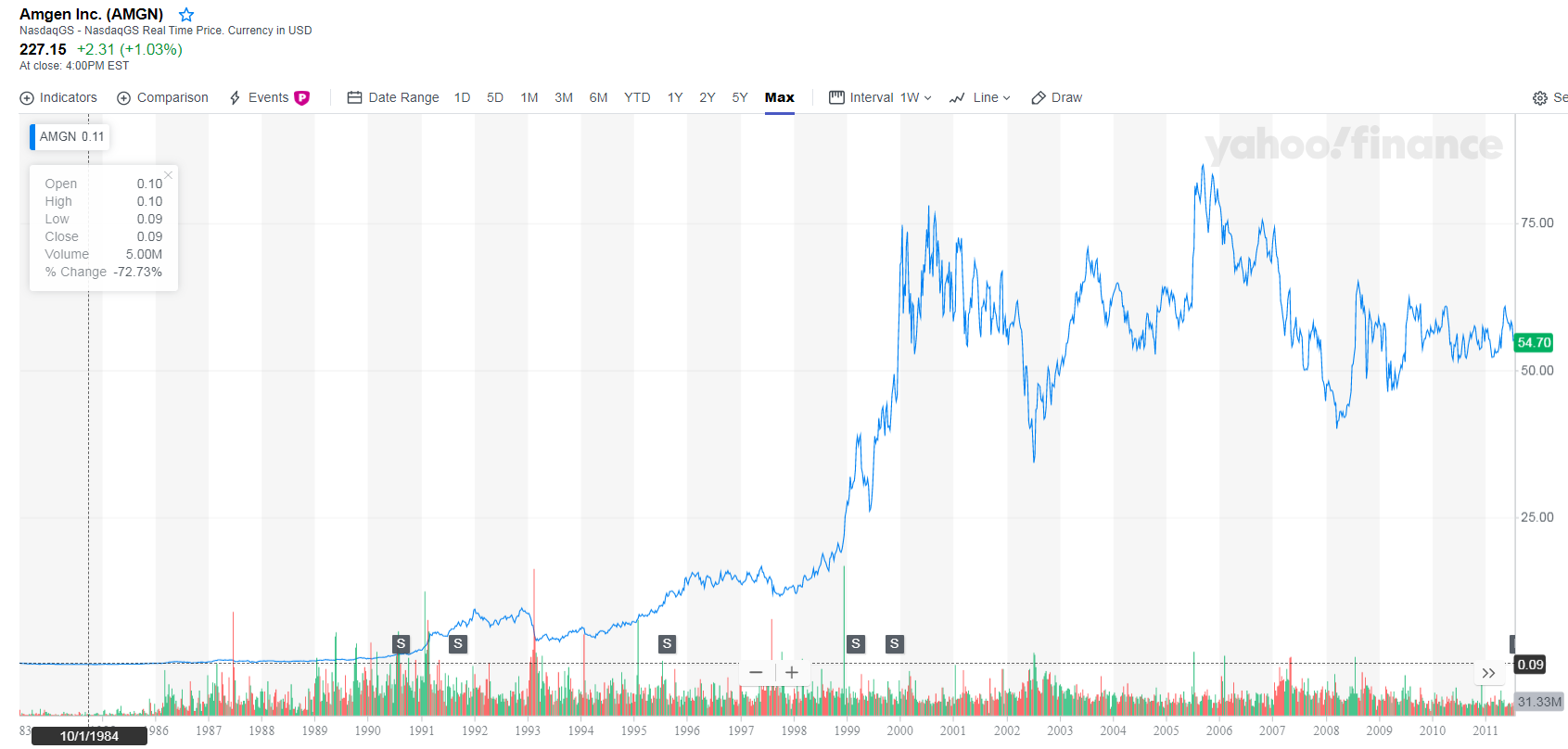### ECON 315 Financial Econometrics and Time Series Analysis - Spring 2020

This course is an introduction to data analysis and econometric modeling using applications in finance and time series. The course uses concepts from microeconomics, finance, mathematical optimization, data analysis, probability models, statistical analysis, and econometrics. The course will be 16 weeks long. Each week consists of one 150 minutes lecture. Finance topics include asset return calculations, risk and performance measures, portfolio theory, index models, and applied time series analysis. Quantitative methods involve basic matrix algebra. Statistical topics include probabilities, expectation, joint distributions, covariance, normal distribution, sampling distributions, estimation and hypothesis testing, data analysis, linear regression, time series methods and simulations. There will be weekly frequent homework assignments requiring STATA programming. Students will work independently and periodically in groups to complete problem sets and group projects. Students will be graded on quizzes/problem sets, midterm and final exams. The course qualifies for all MSE tracks.